# How to VLOOKUP from Another Sheet in Google Sheets: Vlookup Between Two Sheets

VLOOKUP From Another Sheet: The full form of the VLOOKUP function is Vertical lookup. The VLOOKUP function is one of the most useful features which helps to analyse data in one go. Also, the VLOOKUP function helps to save a lot of time which can call data from other sheets and workbooks as well.

The main feature of the VLOOKUP function is to lookup and fetch specific numbers from large amounts of data promptly. Also, It’s easier to keep your data spruce when you can utilise it across numerous spreadsheets, and it automatically updates when there are changes between Google Sheets. On this page, let’s discuss everything about the VLOOKUP function and its feature in detail. Also, don’t miss important VLOOKUP Formulas & Google Sheet Tricks at the end of the article.

## VLOOKUP Formula Syntax

The syntax of the VLOOKUP formula is explained below:

VLOOKUP(search_key, range, index, [is_sorted])

• search_key: It represents the key value or unique identification that you want to look for.
This can be a value or a reference to a cell that consists of value.
• range: The VLOOKUP function should search inside this range of cells (in the source table). Always double-check that this range includes both the column containing the search key and the column containing the value to be retrieved. VLOOKUP always looks for the search key in the first column of the range.
• index: It’s the number of the column in the range where the appropriate value (the one in the same row as search key) should be found. As a result, the range’s first column has an index of 1, the second column has an index of 2, and so on.
• is_sorted: This is optional which can be either TRUE or FALSE.

### VLOOKUP Formula to Fetch Value from Different Sheet

The formula to fetch a value from different sheets into one sheet are given below:

=vlookup(search_key,importrange(“{sheetsURL}”,“{sheet name}!{cell range}”),index,is_sorted)

### VLOOKUP from Another Worksheet in the Same Workbook

Let’s understand how to use the VLOOKUP function with the help of a simple example. In the given example, we have two different workbooks- Employee details and salary details as shown below.

Employee Details Workbook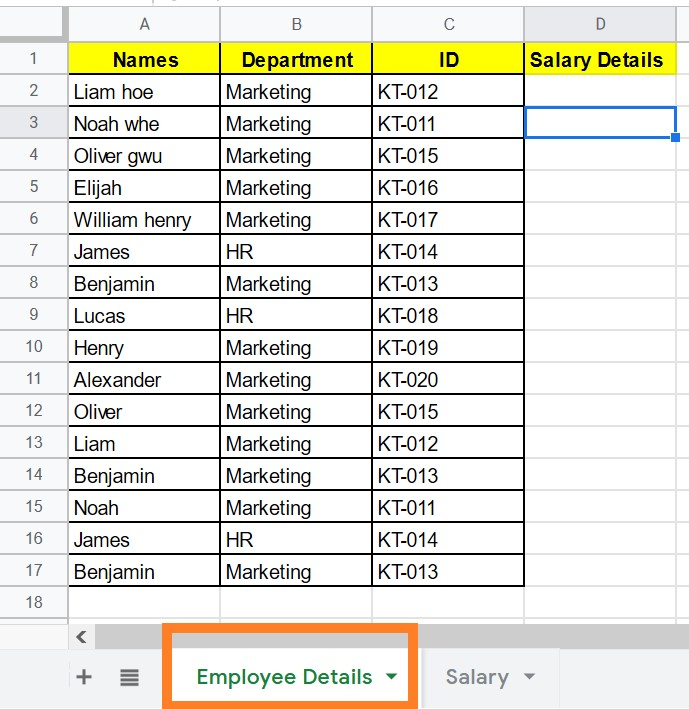Salary Details Workbook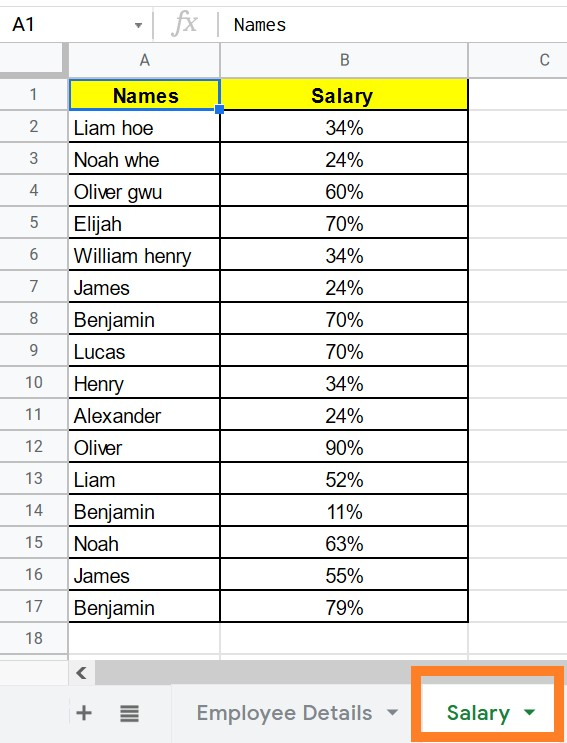Now we have to call the salary details into the employee details tab. To call the same, follow the steps listed below.

• Step 1: Select the cell where you have to call the data.
• Step 2: Now enter the formula “=Vlookup(A2,Salary!A2:C17,2,false).”
• Step 3: Click on “Enter“.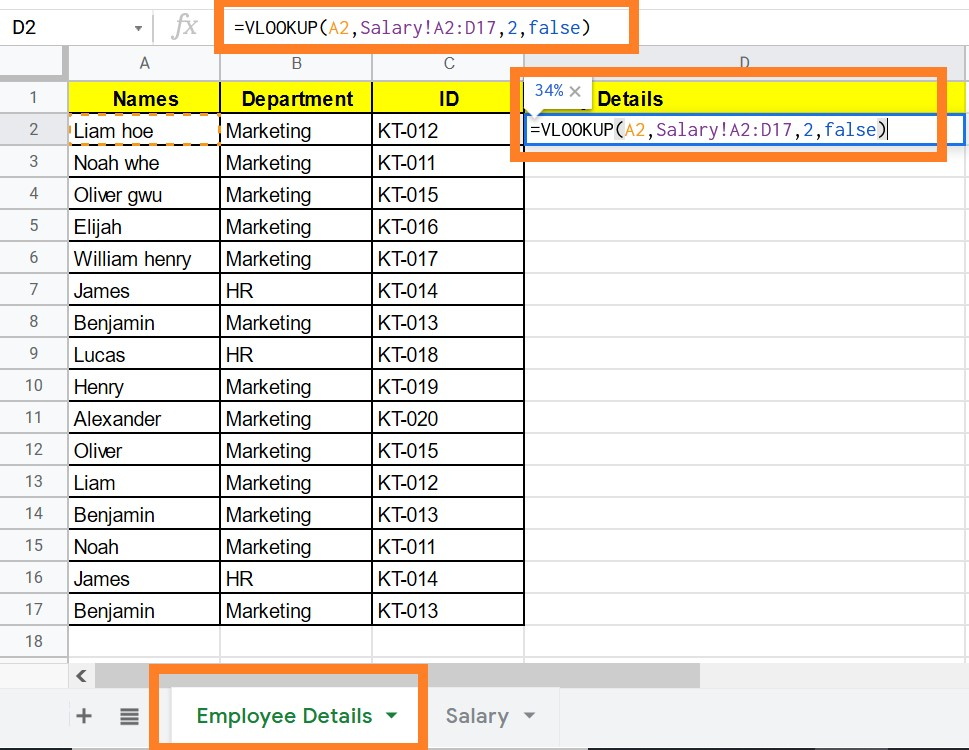• Step 4: Drag the same formula for all the cells.
• Step 5: Now you will see, the Employee Details tab fetching the Salary details.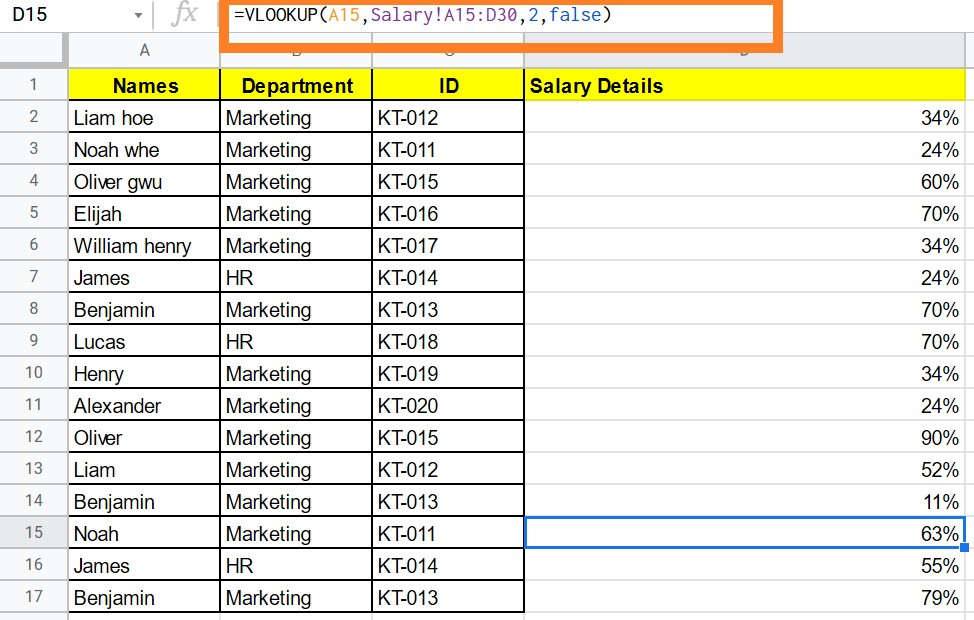### VLOOKUP from Another Worksheet in a Different Workbook

This process is a bit complicated. Let’s consider the same example. Now we have only Employee name, ID and their department. Now we will have to call the employee salary details from the different Google sheets called Salary as shown below.

Follow the steps listed below to VLOOKUP from another worksheet in a different workbook.

• Step 1: Select the cell where you would like the call the data.
• Step 2: Now enter the formula “=Vlookup(A2,importrange(“G-Sheet URL”,”Sheet Name!Cell Range”),2,false).” In our case, the formula is: “=Vlookup(A2,importrange(“https://docs.google.com/spreadsheets/d/1GfuGTaqn_hE7rjksx-ddwQ2WvQRcRng5pLeexZghXlo/”,“Salary!A2:C17”),2,false)
• Step 3: Click on “Enter“.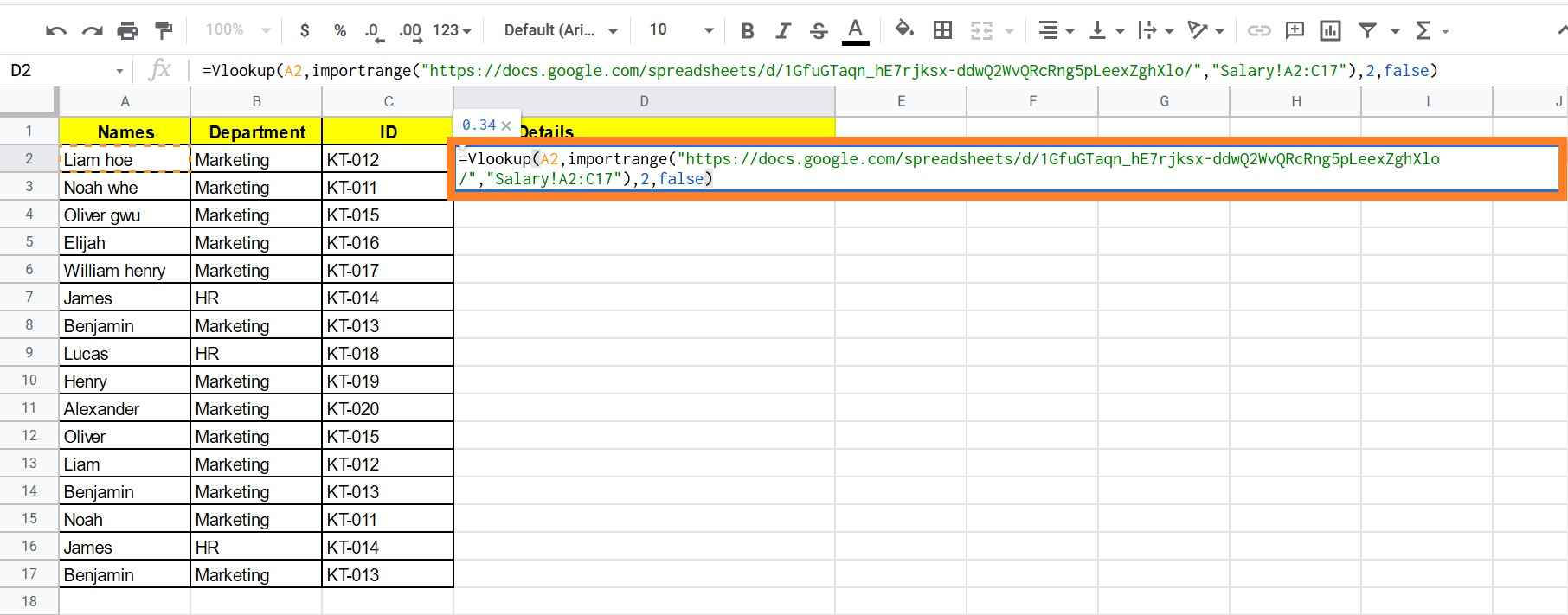• Step 4: Now drag the formula to all the cells.
• Step 5: You will see the sheet fetching the values.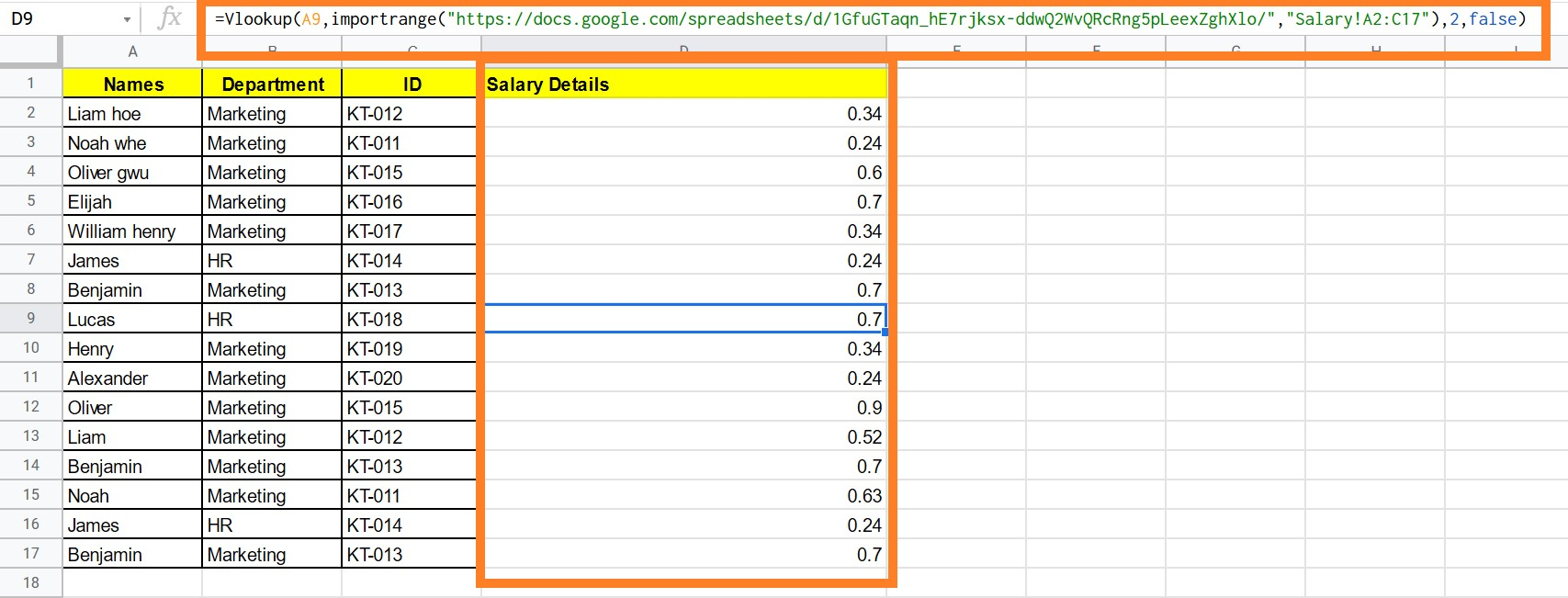### Tips to use VLOOKUP Function

1. Make sure you apply the specific cell range. If the cell range has been mistyped, the Google Sheet will show the REF error.
2. When you are trying to VLookup from one spreadsheet to another spreadsheet, then make sure you have access to the same. If you don’t have access then, the G-Sheet will throw the error.
3. To ease the process, use conditional statements which prevent unnecessary calls.
4. The three important values which need to set in the Vlookup reference are:
• Workbook URL {sheetsURL}
• Sheet Page {sheet name}
• Cell Range {cell range}